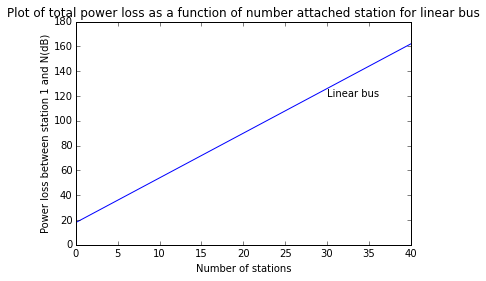Chapter 13: Optical networks¶

Example 13.1, Page Number: 464¶

In :
import math
import numpy as np

#variable declaration
N=np.array([5,10,50])                      #number of station
alpha = 0.4                                #attanuation (dB/km)
L_tap = 10                                 #coupling loss (dB)
L_thru = 0.9                               #coupler throughput(dB)
Li = 0.5                                   #intrinsic coupler loss(dB)
Lc = 1.0                                   #coupler to fiber loss(dB)

#calculation
fiber_Loss = alpha *L                                                   #fiber loss(dB)
Pbudget = N*(alpha*L+2*Lc+Li+ L_thru)-alpha*L-2*L_thru +2* L_tap        #power budget(dB)

#result
print "Fiber loss at 500m =",fiber_Loss,"dB"
print "Power budget of three stations 5, 10, 50 respectively = ",Pbudget,"dB",Pbudget,"dB",Pbudget,"dB"
Fiber loss at 500m = 0.2 dB
Power budget of three stations 5, 10, 50 respectively =  36.0 dB 54.0 dB 198.0 dB

Example 13.2, Page Number: 465¶

In :
import math
import matplotlib.pyplot as plt
import numpy as np
import scipy as sp
from scipy import special

%matplotlib inline

#variable declaration
alpha = 0.4                                #attanuation (dB/km)
L_tap = 10                                 #coupling loss (dB)
L_thru = 0.9                               #coupler throughput(dB)
Li = 0.5                                   #intrinsic coupler loss(dB)
Lc = 1.0                                   #coupler to fiber loss(dB)
Pbudget_LED = 38                           #power loss of LED
Pbudget_LASER = 51                         #power loss of LASER

#calculation
N_LED = (Pbudget_LED + alpha*L-2*L_thru-2*L_tap)/(alpha*L+2*Lc+Li+L_thru)
N_LASER = (Pbudget_LASER + alpha*L-2*L_thru-2*L_tap)/(alpha*L+2*Lc+Li+L_thru)

#result
print "Number of stations allowed for given loss of 38 dB with LED source =",round(N_LED,0)
print "Number of stations allowed for given loss of 51 dB with LASER source =",round(N_LASER,0)

#plot
x1=arange(0.0, 50.0, 10.0)
Pbudget1 = x1*(alpha*L+2*Lc+Li+ L_thru)-alpha*L-2*L_thru +2* L_tap
plot(x1,Pbudget1)
title('Plot of total power loss as a function of number attached station for linear bus')
ylabel('Power loss between station 1 and N(dB)')
xlabel('Number of stations')
text(30,120,'Linear bus')
Number of stations allowed for given loss of 38 dB with LED source = 5.0
Number of stations allowed for given loss of 51 dB with LASER source = 8.0
Out:
<matplotlib.text.Text at 0xa859828>Example 13.3, Page Number: 465¶

In :
import math
import numpy as np

#variable declaration
N=np.array([5,10])                         #number of station
alpha = 0.4                                #attanuation (dB/km)
L_thru = 0.9                               #coupler throughput(dB)
Li = 0.5                                   #intrinsic coupler loss(dB)
Lc = 1.0                                   #coupler to fiber loss(dB)

#calculation
DR = (N-2)*(alpha*L+2*Lc+Li+L_thru)           #dynamic range(dB)

#result
print "Dynamic range for number of station 5 = ",DR,"dB"
print "Dynamic range for number of station 10 = ",DR,"dB"
Dynamic range for number of station 5 =  10.8 dB
Dynamic range for number of station 10 =  28.8 dB

Example 13.4, Page Number: 466¶

In :
import math

#variable declaration
N1=10                                      #number of station
N2=50                                      #number of station
alpha = 0.4                                #attanuation (dB/km)
Lc = 1.0                                   #coupler to fiber loss(dB)
Lexcess1 = 0.75                            #excess loss for 10 station
Lexcess2 = 1.25                            #excess loss for 50 station

#calculation
Ps_Pr1 = Lexcess1+alpha*2*L+2*Lc+10*math.log10(N1)              #power margin for 10 station(dB)
Ps_Pr2 = Lexcess2+alpha*2*L+2*Lc+10*math.log10(N2)              #power margin for 50 station(dB)

#result
print "Power margin between transmitter and receiver for 10 station =",round(Ps_Pr1,1),"dB"
print "Power margin between transmitter and receiver for 50 station =",round(Ps_Pr2,1),"dB"
Power margin between transmitter and receiver for 10 station = 13.2 dB
Power margin between transmitter and receiver for 50 station = 20.6 dB

Example 13.5, Page Number: 477¶

In :
import math

#variable declaration
L_OM2 = 40                                  #Length of OM2 fiber(meter)
L_OM3 = 100                                 #Length of OM3 fiber(meter)
BW_OM2 = 500*10**6                          #bandwidth of OM2 fiber(MHz)
BW_OM3 = 2000*10**6                         #bandwidth of OM3 fiber(MHz)

#calculation
Lmax = L_OM2*(BW_OM3/BW_OM2)+L_OM3          #Maximum link length(meter)

#result
print "Maximum link length = ",Lmax,"m"
Maximum link length =  260 m